matlab傅立叶图像处理_matlab图像处理傅里叶逆变换 - CSDN
• ## matlab数字图像的傅立叶变换实验

万次阅读 多人点赞 2016-11-11 11:53:45
首先读取一幅图像，然后将这幅图像归一化到0~1之间，然后对图像做二维离散傅立叶变换，然后做快速傅里叶变换，即直流分量移到频谱中心，让正半轴部分和负半轴部分的图像分别关于各自的中心对称，然后取傅立叶变换的...
实验三  图像的傅立叶变换
1.启动MATLAB程序，读入一幅图像；对图像做FFT。使用’subplot’命令，同时显示原始图像其频谱图；
1.1实验过程：
首先读取一幅图像，然后将这幅图像归一化到0~1之间，然后对图像做二维离散傅立叶变换，然后做快速傅里叶变换，即直流分量移到频谱中心，让正半轴部分和负半轴部分的图像分别关于各自的中心对称，然后取傅立叶变换的实部，然后做频谱对数变换，就得到原图像的频谱图，最后将原图像和对应的频谱图显示出来。
1.2代码及图像
F=fft2(im2double(I)); %FFT
F=fftshift(F); %FFT频谱平移
F=real(F);
T=log(F+1); %频谱对数变换
subplot(1,2,1),imshow(I),title('原始图像');
subplot(1,2,2),imshow(T,[]),title('原始图像其频谱图');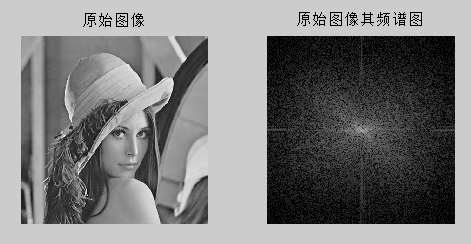2. 读入一幅图像，分别为图像添加椒盐、高斯噪声，做FFT变换。使用’subplot’命令，将原始图像、原始图像频谱图、添加噪声后的图像，以及噪声图像的频谱图同时显示出来。
2.1实验过程
得到原始图像的频谱图的方法同上，然后对原图像模拟叠加密度为0.04的椒盐噪声，然后添加模拟均值为0方差为0.02的高斯噪声，然后得到噪声图像的频谱图，方法同上，最后显示所有图像。
2.2代码及图像
F=fft2(im2double(I)); %FFT
F=fftshift(F); %FFT频谱平移
F=real(F);
T=log(F+1); %频谱对数变换
subplot(3,2,1),imshow(I),title('原始图像');
subplot(3,2,2),imshow(T,[]),title('原始图像其频谱图');
S=imnoise(I,'salt & pepper', 0.04); %模拟叠加密度为0.04的椒盐噪声
K=fft2(im2double(S)); %FFT
K=fftshift(K); %FFT频谱平移
K=real(K);
T=log(K+1); %频谱对数变换
subplot(3,2,3),imshow(S),title('添加椒盐噪声后的图像');
subplot(3,2,4),imshow(T,[]),title('椒盐噪声频谱图');
G=imnoise(I,'gaussian',0,0.02);%模拟均值为0方差为0.02的高斯噪声，
H=fft2(im2double(G)); %FFT
H=fftshift(H); %FFT频谱平移
H=real(H);
T=log(H+1); %频谱对数变换
subplot(3,2,5),imshow(G),title('添加高斯噪声后的图像');
subplot(3,2,6),imshow(T,[]),title('高斯噪声频谱图');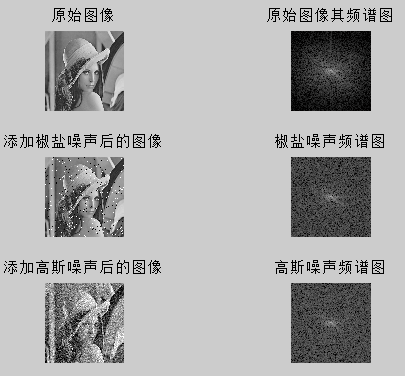3. 读入一幅图像，对图像分别进行高斯低通、巴特沃兹低通、高斯高通和巴特沃兹高通频域滤波，比较其锐化和平滑效果；
3.1实验过程
对于高斯低通频域滤波，首先求原图像的频谱图，然后根据二维高斯低通滤波器(GLPF)定义，对频谱图做高斯低通滤波，使低频通过而使高频衰减，最后做快速傅里叶逆变换，结果发现滤波后的图像变模糊，比原始图像减少尖锐的细节部分而突出平滑过渡部分；对于巴特沃兹低通频域滤波，然后根据二级巴特沃思低通滤波器(BLPF)定义，对频谱图做巴特沃兹低通滤波，使低频通过而使高频衰减，最后做快速傅里叶逆变换，结果发现滤波后的图像变模糊，比原始图像减少尖锐的细节部分而突出平滑过渡部分；经对比图像后发现，经过巴特沃思低通滤波的图像比经过高斯低通频域滤波的图像更平滑。
对于高斯高通频域滤波，首先求原图像的频谱图，然后根据截频距原点为D0的高斯高通滤波器(GHPF)定义，对频谱图做高斯高通滤波，使高频通过而使低频衰减，最后做快速傅里叶逆变换，结果发现滤波后的图像变锐化，比原始图像减少平滑过渡而突出边缘等细节部分；对于巴特沃兹高通频域滤波，然后根据二阶且截至频率距原点的距离为D0的巴特沃思高通滤波器(BHPF)定义，对频谱图做巴特沃兹高通滤波，使高频通过而使低频衰减，最后做快速傅里叶逆变换，结果发现滤波后的图像变锐化，比原始图像减少平滑过渡而突出边缘等细节部分；经对比图像后发现，经过高斯低通滤波的图像比经过高斯低通巴特沃思低通频域滤波的图像更平滑。
3.2代码及图像
%高斯低通频域滤波
subplot(1,2,1),imshow(I),title('原始图像');
I=double(I);
S=fftshift(fft2(I));
[M,N]=size(S);
n=2;
d0=30; %GLPF滤波，d0=5，15，30（程序中以d0=30为例）
n1=floor(M/2);
n2=floor(N/2);
for i=1:M
for j=1:N
d=sqrt((i-n1)^2+(j-n2)^2);
h=1*exp(-1/2*(d^2/d0^2));
S(i,j)=h*S(i,j);
end
end
S=ifftshift(S);
S=uint8(real(ifft2(S)));
subplot(1,2,2),imshow(S),title('高斯低通滤波图像');
%巴特沃斯低通频域滤波
subplot(1,2,1),imshow(I),title('原始图像');
F=double(I);                % 数据类型转换，MATLAB不支持图像的无符号整型的计算
G=fft2(F);                    % 傅立叶变换
G=fftshift(G);                 % 转换数据矩阵
[M,N]=size(G);
nn=2;                       % 二阶巴特沃斯(Butterworth)低通滤波器
d0=30;                      %截止频率为30
m=fix(M/2); n=fix(N/2);
for i=1:M
for j=1:N
d=sqrt((i-m)^2+(j-n)^2);
h=1/(1+0.414*(d/d0)^(2*nn));         % 计算低通滤波器传递函数
result(i,j)=h*G(i,j);
end
end
result=ifftshift(result);
Y2=ifft2(result);
Y3=uint8(real(Y2));
subplot(1,2,2),imshow(Y3),title('巴特沃斯低通滤波')
%巴特沃斯高通频域滤波
subplot(121),imshow(I); title('原始图像');
F=double(I);% 数据类型转换，MATLAB不支持图像的无符号整型的计算
G=fft2(F);% 傅立叶变换
G=fftshift(G);%转换数据矩阵
[M,N]=size(G);
nn=2;% 二阶巴特沃斯(Butterworth)高通滤波器
d0=30;
m=fix(M/2);n=fix(N/2);
for  i=1:M
for j=1:N
d=sqrt((i-m)^2+(j-n)^2);
if (d==0)
h=0;
else
h=1/(1+0.414*(d0/d)^(2*nn));% 计算传递函数
end
result(i,j)=h*G(i,j);
end
end
result=ifftshift(result);
J2=ifft2(result);
J3=uint8(real(J2));
subplot(122),imshow(J3); title('巴特沃斯高通滤波后图像'); % 滤波后图像显示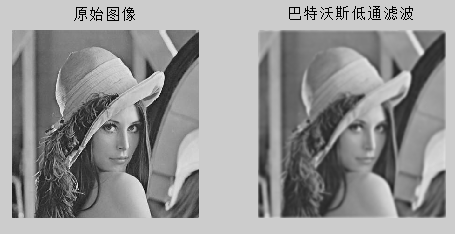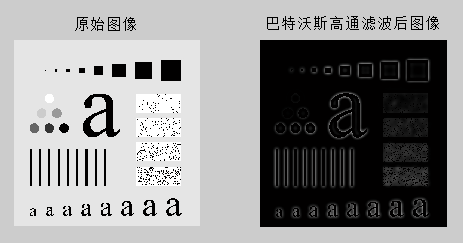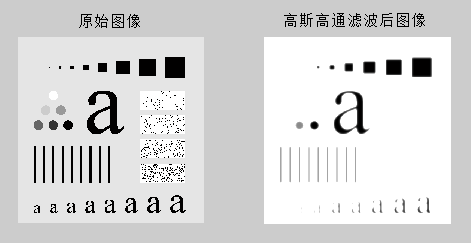展开全文matlab 数字图像处理 傅里叶变换
• matlab图像进行分数傅里叶处理matlab图像进行分数傅里叶处理matlab
• matlab快速傅里叶变换进行数字图像处理,读入一幅灰度图像并实现图像的快速傅立叶变换，求变换后的系数分布。
•傅里叶变换
• ## Matlab对图像进行傅里叶变换实例

万次阅读 多人点赞 2019-04-15 21:05:23
使用matlab对peppers.png图像进行二维傅里叶快速变换及逆变换，从简单的实例中发现问题
一、实验目的
1、了解图像变换的意义和手段；
2、熟悉傅里叶变换的基本性质；
3、熟练掌握FFT的方法与应用；
4、通过实验了解二维频谱的分布特点；
5、通过本实验掌握利用MATLAB编程，实现数字图像的傅里叶变换。
二、实验仪器
1、计算机；
2、MATLAB程序；
3、移动式存储器（软盘、U盘等）；
4、记录用的笔、纸。
三、实验原理
1、应用傅里叶变换进行图像处理
傅里叶变换是线性系统分析的一个有力工具，它能够定量地分析诸如数字化系统、采样点、电子放大器、卷积滤波器、噪音和显示点等的作用。通过实验培养这项技能，将有助于解决大多数图像处理问题。对任何想在工作中有效应用数字图像技术的人来说，把时间用在学习和掌握傅里叶变换上是很有必要的。
2、傅里叶（Fourier）变换的定义
对于二维信号，二维Fourier变换定义为：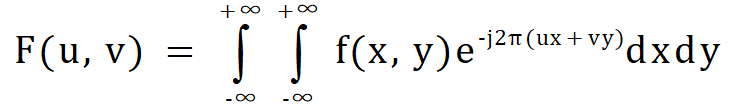逆变换：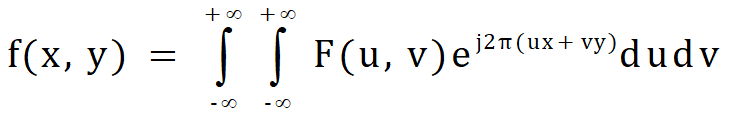二维离散傅里叶变换为：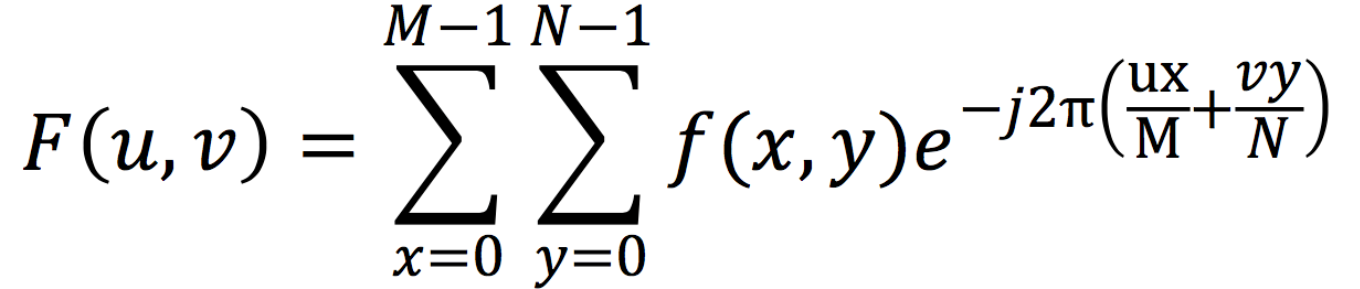逆变换：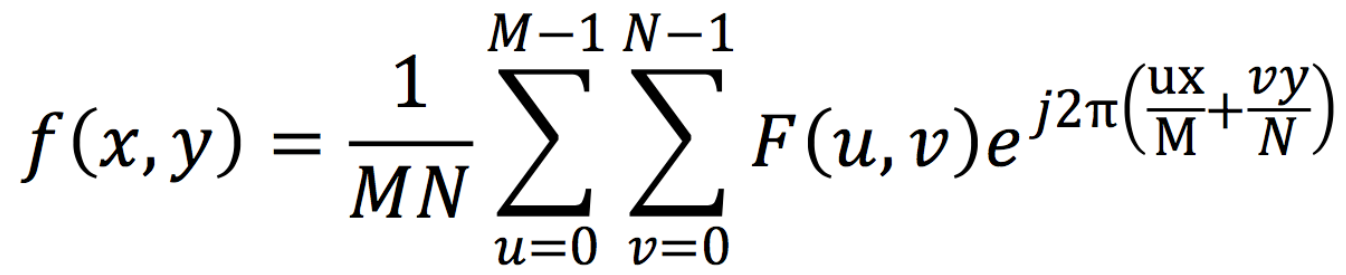图像的傅里叶变换与一维信号的傅里叶变换一样，有快速算法，具体参见参考书目，有关傅里叶变换的快速算法的程序不难找到。实际上，现在有实现傅里叶变换的芯片，可以实时实现傅里叶变换。
3、利用MATLAB软件实现数字图像傅里叶变换、DCT变换的程序。
四、实验步骤
1、打开计算机，安装和启动MATLAB程序；程序组中“work”文件夹中应有待处理的图像文件；
2、利用MATLAB工具箱中的函数绘制FFT频谱显示的函数；
3、a）调入、显示图像；
b）对这图像做FFT、DCT并利用自编的函数显示其频谱；
c）讨论不同的图像内容与FFT、DCT频谱之间的对应关系。
4、记录和整理实验报告。
五、实验内容
MATLAB源程序如下：
clear
clc
subplot(2,2,1);imshow(img);title('原图');
f=rgb2gray(img);    %对于RGB图像必须做的一步，也可以用im2double函数
F=fft2(f);          %傅里叶变换
F1=log(abs(F)+1);   %取模并进行缩放
subplot(2,2,2);imshow(F1,[]);title('傅里叶变换频谱图');
Fs=fftshift(F);      %将频谱图中零频率成分移动至频谱图中心
S=log(abs(Fs)+1);    %取模并进行缩放
subplot(2,2,3);imshow(S,[]);title('频移后的频谱图');
fr=real(ifft2(ifftshift(Fs)));  %频率域反变换到空间域，并取实部
ret=im2uint8(mat2gray(fr));    %更改图像类型
subplot(2,2,4);imshow(ret),title('逆傅里叶变换');

六、实验结果
在MATLAB中运行后，实验结果如图：
在Figure1中，左上角显示的是读入MATLAB程序的原图片，右上角显示的是经过二维傅里叶快速变换后的频谱图像，左下角显示的是将频谱中零频率成分移至矩阵的中心后的频谱图像。右下角显示的是二维傅里叶逆变换后的图像。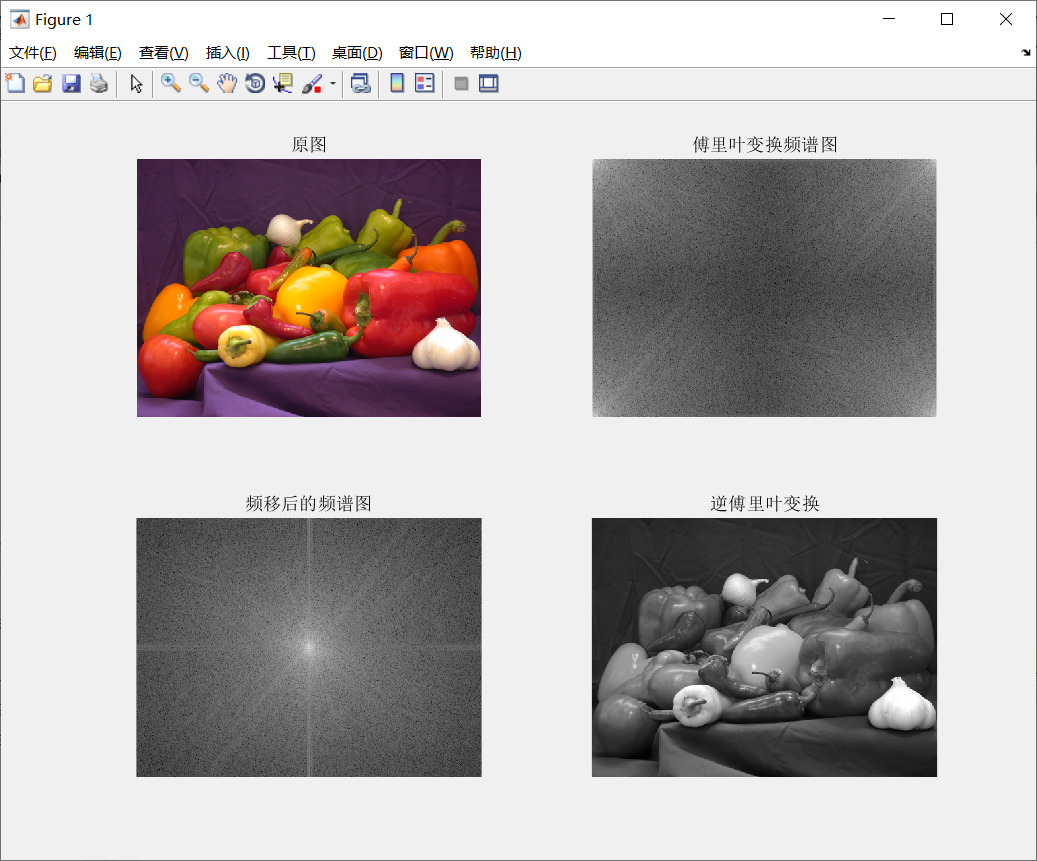图1 MATLAB程序运行后结果
七、实验中遇到的问题及对实验过程的思考
1、关于imshow函数：
使用imshow函数显示图像时要注意图像矩阵类型，当图像是double类型时要使用imshow（I,[]）来根据数据矩阵的数值范围自动设置灰度图像显示范围。
详细可参考matlab官网参考文档：显示图像-MATLAB imshow-MathWorks中国
2、关于运行时可能出现的警告：
如果图像进行傅里叶转换后立即用imshow函数显示，则在命令行可能会显示：Warning: Displaying real part of complex input（警告: 显示复数输入项的实部）。这是因为经过傅里叶变换后的图像矩阵大多是复数矩阵，包含实部和虚部。此时如要显示图像则需要先用abs取复数矩阵的模，再进行显示。
取模后图像矩阵的数值一般会很大，直接用imshow函数是无法显示的，此时可以用log函数取其对数，如log(abs(F)+1)，这样就可以对频谱进行缩放。至于为什么用log(F+1)，如下图所示，对于（0，1）之间的x值经过取对数后会变成负值，而log（x+1）则将所有的x值映射到正数范围内。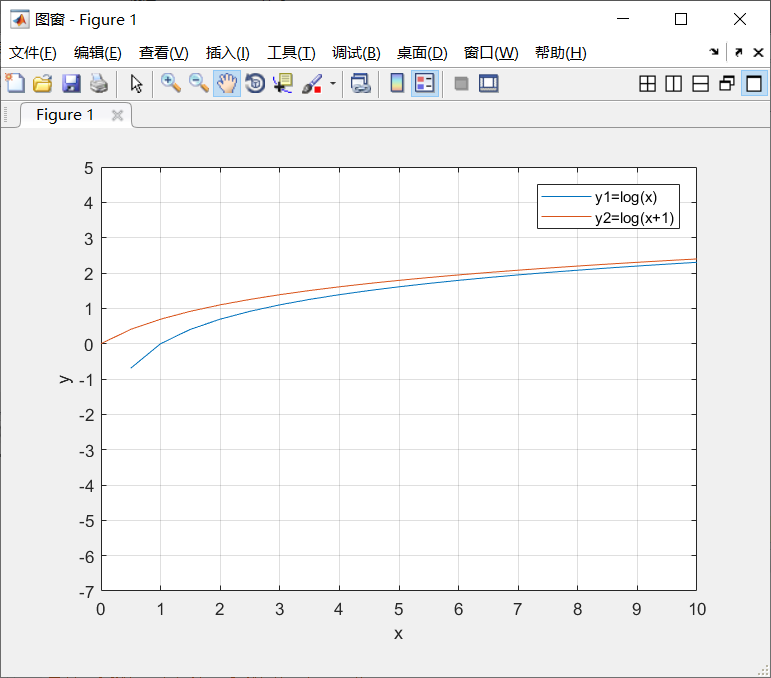图2 log
3、关于开头im2double和rgb2gray函数的使用：
对于RGB真彩图像来说，读入后是以三维矩阵形式存储的，如果此时直接进行傅里叶变换，则频谱图会显示为一片空白或者是密密麻麻的。所以在对RGB图像进行傅里叶变换前要进行类型转换，可以使用im2double将其转换为双精度型（图像运算很少有整型的，所以保守起见不管什么图像都先用这个函数进行转换），或者使用rgb2gray将其转换为灰度图像。
使用不同的函数其变换效果也是不一样的。如使用im2double，进行傅里叶变换后其频谱图呈白色基调，使用rgb2gray其频谱图呈灰色基调。
4、关于最后使用im2uint8转换图像类型：
这一步是为了将逆转换得到的矩阵先转换为灰度图像（mat2gray），再将图像类型转换为uint8。其实这一步的调用与否与开头两个类型转换函数的使用有关，如果在开头就已经将RGB图像转为灰度图像，则此步骤可省去，直接显示经逆转换的图像。如果开头调用im2double，此步省略后可显示出彩色图像。当然此步骤在不同方面发挥着不同作用，取的灰度图像可以为后续操作提供基础。
更多函数信息可参考matlab官网    MATLAB-函数
如有错漏之处敬请指正


展开全文matlab
• 代码如下： clear; clc; img=imread('test.jpg'); img=rgb2gray(img); ... %傅里叶变换 f=fftshift(f); %使图像对称 r=real(f); %求图像频域实部 i=imag(f); %求图像频域虚部 margin=log(abs(f));

使用matlab中自带的fft等相关的函数进行操作。

代码如下：

clear;
clc;

img=rgb2gray(img);

f=fft2(img);        %傅里叶变换
f=fftshift(f);      %使图像对称
r=real(f);          %求图像频域实部
i=imag(f);          %求图像频域虚部
margin=log(abs(f));      %图像幅度谱，加log便于显示
phase=log(angle(f)*180/pi);     %图像相位谱
l=log(f);

subplot(2,2,1),imshow(img),title('源图像');
subplot(2,2,2),imshow(l,[]),title('频谱');
subplot(2,2,3),imshow(margin,[]),title('幅度谱');
subplot(2,2,4),imshow(phase,[]),title('相位谱');


展开全文图像处理
• ## Matlab对图像的傅里叶变换初探

万次阅读 多人点赞 2020-01-17 20:41:57
图像： clc; clear; I=imread('1.jpg'); % 读取图像 I=rgb2gray(I); % RGB图像转换为灰度图 - （可试试去掉然后做对比） I=im2double(I); % 图像转换为double，此时图像的数值范围由原来的【0,255】...
图像频率的概念
图像的频率指的是空间频率，它和我们认知的物理频率是不同的，因此，要理解图像频率，就要将两者的定义脱离开。
图像可以看成是一个定义在二维平面上的信号，该信号的幅值对应像素的灰度（彩色图像对应 RGB 三个分量）。
如果仅仅考虑一帧图像的某一行像素，那么，可以看成是一维空间的信号。这种信号和我们常见的时域信号是很相似的，只不过时域信号是定义在时间域上的，而图像信号是定义在空间域的。
因此，图像的频率被称为空间频率，空间频率是指单位长度内亮度做周期性变化的次数，它反映了图像的像素灰度在空间中的变化情况，从傅里叶频谱上可以看到明暗不一的亮点，反映的就是某点与邻域间的差异程度。
举个例子，一帧图像的背景或者变化缓慢的区域，也就是灰度值分布比较平坦，那么，低频分量就比较强。图像的边缘、细节以及噪声的像素灰度在空间的变化非常剧烈。因此为高频分量。
根据傅里叶变换理论，频域中的频谱值都是由整幅图像计算出来的，因此，整个频谱范围内，低频分量集中在四个角，且其它地方的值只可能比这个值小。

图像变换
原图像：clc;
clear;
I=rgb2gray(I);          % RGB图像转换为灰度图 - （可试试去掉然后做对比）
I=im2double(I);         % 图像转换为double，此时图像的数值范围由原来的【0,255】转化为【0,1】 -（可试试去掉然后做对比）
F=fft2(I);              % 进行傅里叶变换
F=fftshift(F);          % 傅里叶变换后的图像进行象限转换
F=abs(F);               % 求傅里叶变换的模。变换后的结果是一个复数，有实部和虚部，abs求其模。 - 并且如果没这一句的会有警告！
T=log(F+1);             % 上一步得到了傅里叶变换的模，但是这个值一般是非常大的，为了更好的观察图像我们对其进行缩放。
figure;                 % 创建图像
imshow(T,[]);           % imshow显示的只有0~1的值。尽管我们进行了缩放，凡是还是部分值大于1，后面加了[]是为了大于1的值相应的映射到0~1这个区间里！

FT后的图像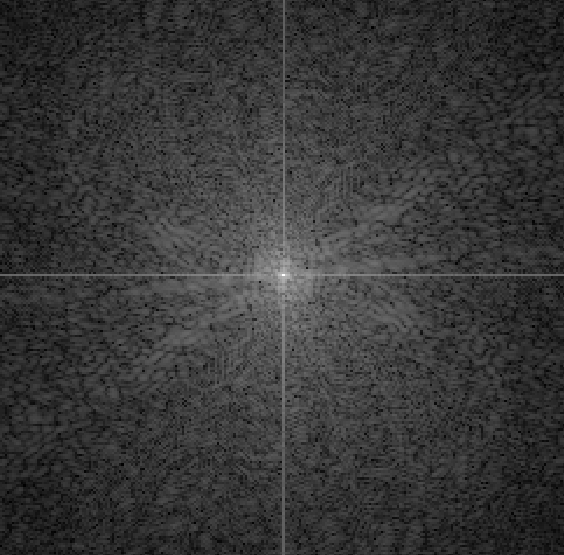FT变换的更进一步探讨
clc;
clear;
figure;                 % 创建一个图像句柄
subplot(2,2,1);         % 对应显示的子图位置
imshow(I);              % 原图显示
title('原图');
I=rgb2gray(I);          % RGB图像转换为灰度图 - （可试试去掉然后做对比）
subplot(2,2,2)          % 对应显示的子图位置
imhist(I);              % 显示图像灰度直方图
title('灰度直方图');
%I=im2double(I);         % 图像转换为double，此时图像的数值范围由原来的【0,255】转化为【0,1】 所以可以理解为二值化-（可试试去掉然后做对比）
F=fft2(I);              % 进行傅里叶变换
IF = F;                 % 句柄备份为了傅里叶逆变换
F=fftshift(F);          % 傅里叶变换后的图像进行象限转换
F=abs(F);               % 求傅里叶变换的模。变换后的结果是一个复数，有实部和虚部，abs求其模。 - 并且如果没这一句的会有警告！
T=log(F+1);             % 上一步得到了傅里叶变换的模，但是这个值一般是非常大的，为了更好的观察图像我们对其进行缩放。
subplot(2,2,3)          % 对应显示的子图位置
imshow(T,[]);           % imshow显示的只有0~1的值。尽管我们进行了缩放，凡是还是部分值大于1，后面加了[]是为了大于1的值相应的映射到0~1这个区间里！
title('傅里叶变换后的频谱图');
R=uint8(real(ifft2(IF)));% 进行IFFT变换使图像复原
subplot(2,2,4)          % 对应显示的子图位置
imshow(R,[]);           % 显示傅里叶逆变换后的图像
title('傅里叶逆变换后的复原图像');
suptitle('FT相关变换图像');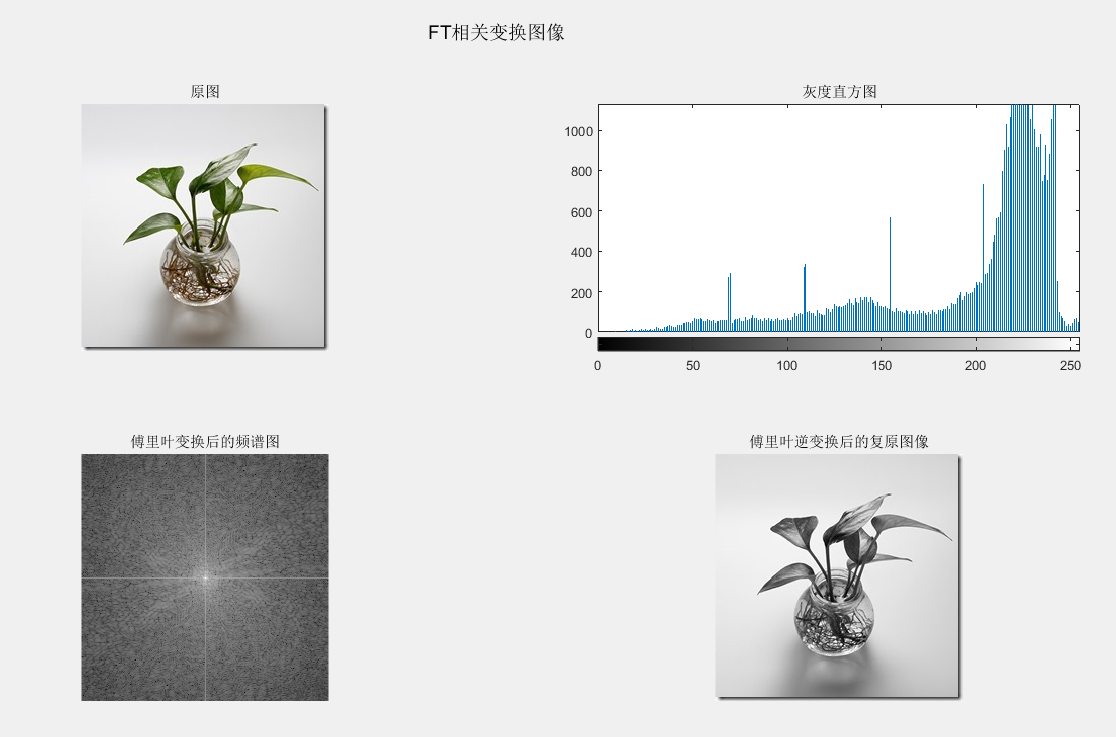把程序I=im2double(I);取消屏蔽后，再来看下对应的图像。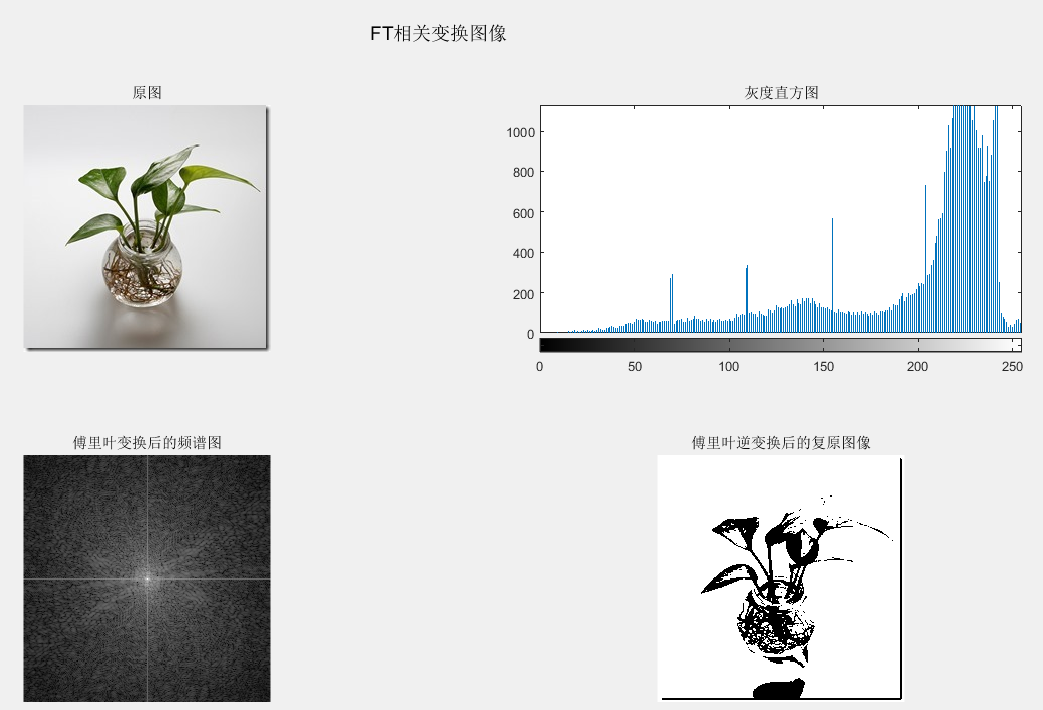也就不难理解这个imdouble,其实就是个二值化函数，把图像进行二值化！
关于几个重要函数这里也再说明下：
FFT函数：FFT（Fast Fourier Transformation）为一阶快速傅里叶变换函数，高速傅里叶变换（FFT）的实质是“分而治之”，利用对称性、周期性和可约性将某些项合并，将DFT序列分解为短序列，降低运算次数，提高运算速度。
IFFT函数：为傅里叶的逆变换，IFFT算法（x）为FFT算法相同（X），除了改变符号，N =长度比例因子（X）。由于FFT、IFFT执行时间取决于变换长度。这是最快的两个权力。这是几乎一样快，只有小素数的长度。它通常是几倍的速度的长度是总理或具有大素数因子。

关于编程中需要注意的细节：
1、imshow(T,[]);
figure;imshow(T,[]);，具体对应到程序中就是ff2或iff2后的图像显示方法了。之所以用imshow(T,[]);而不是imshow(T)。是因为即使经过对数变换后T的取值范围仍然大于[0,1]，比如，maxT=12.23,minT=0.009。
imshow(T)只会显示[0,1]的值，而imshow(T,[]) 会根据灰度图的数值范围来显示图像，相当于将[0.09,12.23]映射到[0,1]显示。
2、经过ff2后的句柄，到imshow显示还得进行中间处理。
总结为：
F = fft2(I);
log(abs(ff2shift(F))+1);
imshow(T, []);


然后就到了感悟环节了
关于傅里叶变换的应用愚钝的我也仅仅知道这不过是冰山一角罢了，这个图像的变换然后再还原什么应用呢？明显，在图像的压缩大有用武之地！
图像显然缩小了，不是吗？


展开全文• https://www.cnblogs.com/h2zZhou/p/8405717.html傅里叶变换
• 全部采用MATLAB语言编程实现，并结合实例对算法程序进行了验证和分析，其中详细讲解了图像及其分类、图像数字化技术、图像处理和分析、颜色空间分析、点运算、图像的代数运算、离散傅里叶变换、快速傅里叶变换、离散...
• 图像处理 二维离散傅里叶变换DFT matlab代码图像处理领域离散傅里叶变换的作用二维离散傅里叶变换二维离散傅里叶变换公式将二维的离散傅里叶变换进行转化将系数转化为矩阵形式注意，从矩阵的乘积i形式可以看出，原来...图像处理代码 matlab 二维离散傅里叶变换
• 其中，详细讲解了图像及其分类、图像数字化技术、图像处理和分析、颜色空间分析、点运算、图像的代数运算、离散傅里叶变换、快速傅里叶变换、离散余弦变换、图像的增强、图像的复原、图像的压缩编码、图像的检测与...
• 第二次终于完全理解了图像处理里面的傅里叶变换，第一次看了最后也没完全弄懂，可能因为当时没有手写程序，所以只是一知半解。 本文关于理论只是粗糙的见解，详细说明可以看上面的参考链接。 关键就是以下几...
• 可以使用 MATLAB傅里叶变换函数和反变换函数。 原理部分 低通滤波器指去除图像中的高频成分，而低通滤波器指去除图像中的高频成分。考虑的有三种低通滤波器：理想滤波器、布特沃斯滤波器和高斯滤波器。理想低通...数字图像 matlab 低通滤波
• 目录1.Objectives:2.Experiment Content：3.Experiment Principle:4.Experiment Steps Result and Conlusion： ...2、 掌握二维 DFT 变换的 MATLAB 程序 3、 空间滤波及频域滤波 2.Experiment Content： 学习使用...计算机视觉
• ## 傅里叶变换及其实现（MATLAB）

万次阅读 多人点赞 2018-07-23 09:55:15
对应于傅里叶变换，频域连续非周期 2. 周期性连续性信号 对应于傅立叶级数，频域离散非周期 3. 非周期离散信号 对应于DTFT（离散时间傅立叶变换），频域连续周期 4. 周期性离散信号 对应于Dmatlab 傅里叶变换
• matlab中对图像进行傅里叶变换和逆变换。注意：m文件中的文件路径是在本人电脑上的路径，用户应修改成在您电脑中图片的绝对路径。谢谢大家的支持！
• matlab数字图像处理实验 1、什么是平滑处理？  首先明白几个名词：  (1)噪声：图像噪声是指存在于图像数据中的不必要的或多余的干扰信息。 1.高斯噪声：高斯噪声是指它的概率密度函数服从高斯分布（即正态...matlab 滤波 图像处理
• 先对图像进行傅里叶变换，再中心化图像。通过高斯高通滤波器的处理后，通过反变换生成新的图像matlab
...